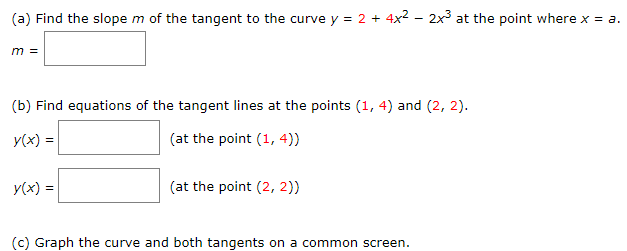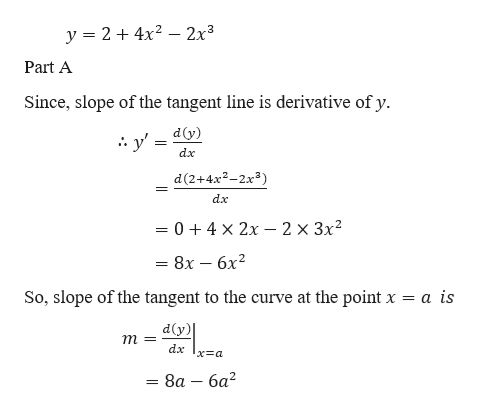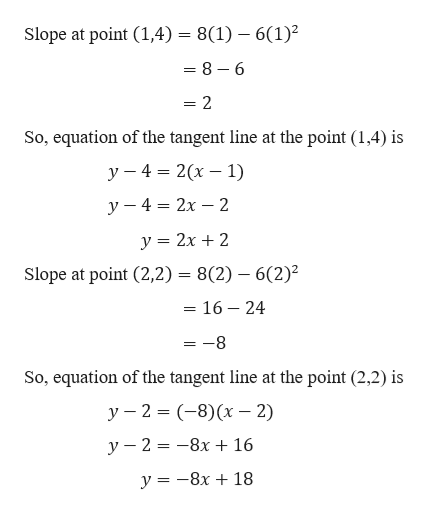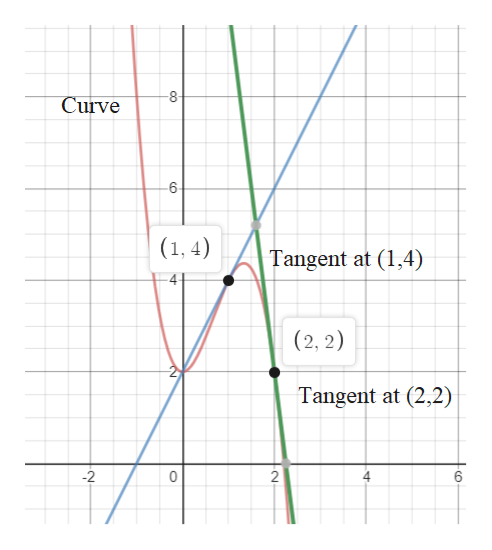# m of the tangent to the curve y2 4x2 - 2x3 at the point where x a(a) Find the slope=m =(b) Find equations of the tangent lines at the points (1, 4) and (2, 2)(at the point (1, 4))y(x)(at the point (2, 2))y(x)=

Question
49 viewshelp_outlineImage Transcriptionclosem of the tangent to the curve y 2 4x2 - 2x3 at the point where x a (a) Find the slope = m = (b) Find equations of the tangent lines at the points (1, 4) and (2, 2) (at the point (1, 4)) y(x) (at the point (2, 2)) y(x)= fullscreen
check_circle

Step 1

Given,help_outlineImage Transcriptionclosey 24x2 2x3 Part A Since, slope of the tangent line is derivative of y y'd) dx d(2+4x2-2x dx = 0 4 x 2x - 2 x 3x2 - 8х — бх? So, slope of the tangent to the curve at the point x a is dy) m= dx xa - 8а — ба? = fullscreen
Step 2

Part bhelp_outlineImage TranscriptioncloseSlope at point (1,4) = 8(1) - 6(1) = 8 - 6 = 2 So, equation of the tangent line at the point (1,4) is y 4 2(x 1) у — 4 %3D 2х — 2 y 2x 2 Slope at point (2,2) 8(2) 6(2) = 16 - 24 -8 So, equation of the tangent line at the point (2,2) is у — 2 %3D (-8)(х — 2) у — 2 3 —8х + 16 у %3D — 8х + 18 fullscreen
Step 3

Graph of the curve and tangent at ...help_outlineImage Transcriptionclose8 Curve 6 (1, 4) Tangent at (1,4) 4 (2, 2) Tangent at (2,2) 2 10 2 fullscreen

### Want to see the full answer?

See Solution

#### Want to see this answer and more?

Solutions are written by subject experts who are available 24/7. Questions are typically answered within 1 hour.*

See Solution
*Response times may vary by subject and question.
Tagged in

### Derivative